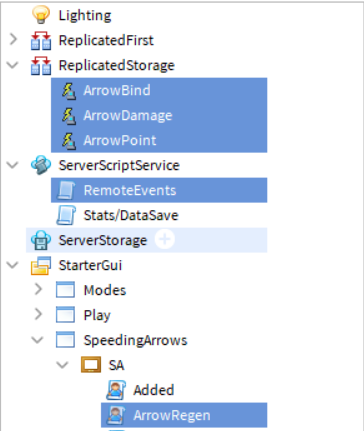# Am I doing something wrong?

So, I tried to make this game, and I ran into this problem. if and elseif ran at the same time and it’s not supposed to. Here’s video footage:

So here’s my local script:

``````local Score = game.Players.LocalPlayer:FindFirstChild("Stats"):WaitForChild("ArrowScore")
local TimeWait = 0.5
local ReplicatedStorage = game:GetService("ReplicatedStorage")
local Point = ReplicatedStorage:WaitForChild("ArrowPoint")
local Damage = ReplicatedStorage:WaitForChild("ArrowDamage")
local Arrow = game.Players.LocalPlayer:WaitForChild("Stats"):WaitForChild("Arrow")

TimeWait = (0.5 + (Score.Value + 1)/(Score.Value + 1) - ((Score.Value + 1)/100))
wait(TimeWait * 2)
if child.Name == "UpArrow" then
if Arrow.Value == 1 then
Point:FireServer(TimeWait)
elseif Arrow.Value ~= 1 then
Damage:FireServer(TimeWait)
end
end
if child.Name == "RightArrow" then
if Arrow.Value == 2 then
Point:FireServer(TimeWait)
elseif Arrow.Value ~= 2 then
Damage:FireServer(TimeWait)
end
end
if child.Name == "DownArrow" then
if Arrow.Value == 3 then
Point:FireServer(TimeWait)
elseif Arrow.Value ~= 3 then
Damage:FireServer(TimeWait)
end
end
if child.Name == "LeftArrow" then
if Arrow.Value == 4 then
Point:FireServer(TimeWait)
elseif Arrow.Value ~= 4 then
Damage:FireServer(TimeWait)
end
end
wait(0.01)
for i = 1,10 do
child.ImageTransparency = child.ImageTransparency + 0.1
wait(0.01)
end
child:Destroy()
end)
``````

and here’s my server script:

``````local ReplicatedStorage = game:GetService("ReplicatedStorage")

-- [UserInputService (SpeedingArrows)] --

local ArrowBind = ReplicatedStorage:WaitForChild("ArrowBind")

ArrowBind.OnServerEvent:Connect(function(player, value, play)
play.Value = value
end)

-- [ArrowPoints (SpeedingArrows)] --

local ArrowPoint = ReplicatedStorage:WaitForChild("ArrowPoint")

local Stats = player:WaitForChild("Stats")
if Stats ~= nil then
local Score = Stats:WaitForChild("ArrowScore")
if Score ~= nil then
print("Point")
end
end
end)

-- [ArrowHealth (SpeedingArrows)] --

local ArrowHealth = ReplicatedStorage:WaitForChild("ArrowDamage")

local Stats = player:WaitForChild("Stats")
if Stats ~= nil then
local Health = Stats:WaitForChild("ArrowHealth")
if Health ~= nil then
print("Damage")
end
end
end)
``````

These are the locations of the scripts and the remote events.It should be: ` ArrowHealth.OnServerEvent:Connect(function(player,ScoreAdding)`